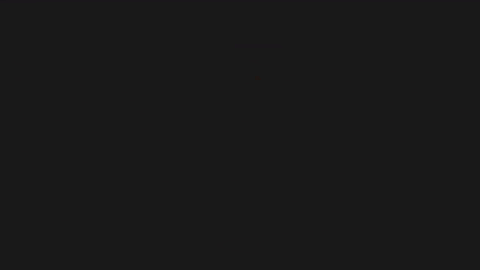DEV Community is a community of 785,073 amazing developers

We're a place where coders share, stay up-to-date and grow their careers.Drawing with Mr. Fourier and Typescript

What if we connect a couple of rotating sticks together and trace the shape they make? We can get something like this.The most interesting part about this is if we get enough sticks we can draw anything we want!

You can try it yourself here. Just draw a closed (that's pretty important) shape and press 'Start'.

This is based on a thing caller Fourier Transform. Basically, every complex function on a set interval can be represented as an infinite sum of exponents:

$f(t) = \sum_{n=-\inf}^{\inf}c_n e^{n 2 \pi i t}, 0 \le t \le 1$

Where

$c_n = \int_0^1 f(t) e^{-n 2 \pi i t}$

But if we look closer we may notice that every element of that sum is actually a rotating vector Cn. So if we manage to represent a shape as a complex function, we could find these vectors (maybe not all, since there is infinite number of them, but we don't need all) and that would mean drawing the shape just by rotating those vectors!

I made a video where I tried to explain this in more detail and code it in TypeScript.

Enjoy!